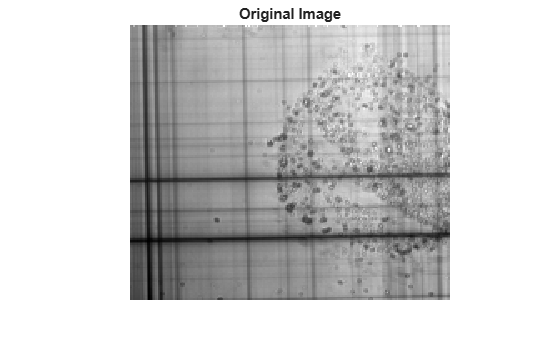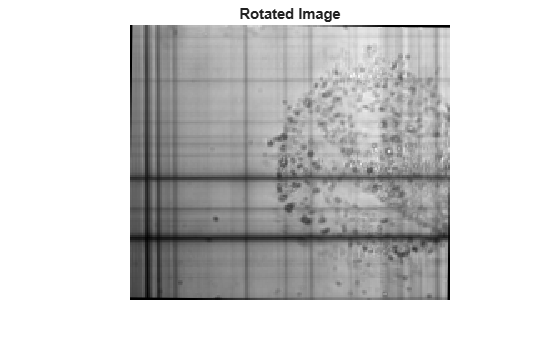# imrotate

## Syntax

``J = imrotate(I,angle)``
``J = imrotate(I,angle,method)``
``J = imrotate(I,angle,method,bbox)``

## Description

example

````J = imrotate(I,angle)` rotates image `I` by `angle` degrees in a counterclockwise direction around its center point. To rotate the image clockwise, specify a negative value for `angle`. `imrotate` makes the output image `J` large enough to contain the entire rotated image. By default, `imrotate` uses nearest neighbor interpolation, setting the values of pixels in `J` that are outside the rotated image to `0`.```

example

````J = imrotate(I,angle,method)` rotates image `I` using the interpolation method specified by `method`.```

example

````J = imrotate(I,angle,method,bbox)` also uses the `bbox` argument to define the size of the output image. You can crop the output to the same size as the input image or return the entire rotated image.```

## Examples

collapse all

Read an image into the workspace, and convert it to a grayscale image.

```I = fitsread('solarspectra.fts'); I = rescale(I);```

Display the original image.

```figure imshow(I) title('Original Image')```Rotate the image 1 degree clockwise to bring it into better horizontal alignment. The example specified bilinear interpolation and requests that the result be cropped to be the same size as the original image.

`J = imrotate(I,-1,'bilinear','crop');`

Display the rotated image.

```figure imshow(J) title('Rotated Image')```## Input Arguments

collapse all

Image to be rotated, specified as a numeric array, logical array, or categorical array.

Data Types: `single` | `double` | `int8` | `int16` | `int32` | `int64` | `uint8` | `uint16` | `uint32` | `uint64` | `logical` | `categorical`

Amount of rotation in degrees, specified as a numeric scalar.

Data Types: `single` | `double` | `int8` | `int16` | `int32` | `int64` | `uint8` | `uint16` | `uint32` | `uint64` | `logical`

Interpolation method, specified as one of the following values:

Value

Description

`'nearest'`

Nearest-neighbor interpolation. The output pixel is assigned the value of the pixel that the point falls within. No other pixels are considered.

Nearest-neighbor interpolation is the only method supported for categorical images.

`'bilinear'`

Bilinear interpolation. The output pixel value is a weighted average of pixels in the nearest 2-by-2 neighborhood.

`'bicubic'`

Bicubic interpolation. The output pixel value is a weighted average of pixels in the nearest 4-by-4 neighborhood.

Note

Bicubic interpolation can produce pixel values outside the original range.

Data Types: `char` | `string`

Bounding box that defines the size of output image, specified as either of the following values:

Value

Description

`'crop'`

Make output image `J` the same size as the input image `I`, cropping the rotated image to fit.

`'loose'`

Make output image `J` large enough to contain the entire rotated image. `J` is larger than `I`.

Data Types: `char` | `string`

## Output Arguments

collapse all

Rotated image, returned as a numeric, logical, or categorical array of the same data type as the input image, `I`.

## Tips

• This function changed in version 9.3 (R2015b). Previous versions of the Image Processing Toolbox™ use different spatial conventions. If you need the same results produced by the previous implementation, use the function `imrotate_old`.

• In some instances, this function takes advantage of hardware optimization for data types `uint8`, `uint16`, `single`, and `double` to run faster.

## Version History

Introduced before R2006a